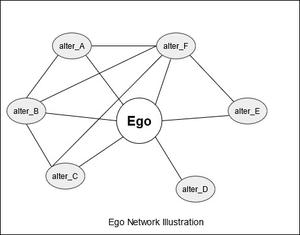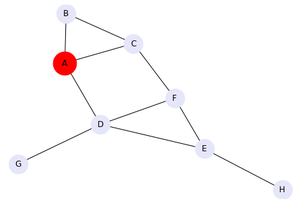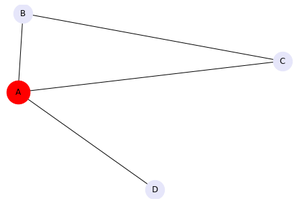GeeksforGeeks App
Open AppBrowser
Continue

# Ego graph Using Networkx in Python

Prerequisite – Graphs, Networkx Basics

Ego network is a special type of network consisting of one central node and all other nodes directly connected to it. The central node is known as ego, while the other surrounding nodes directly connected to it are known as alters. Ego networks are mostly used in analyzing social connections, links, and relationships. The network shown below is an illustration of Ego Network. The central node (ego) is shown as circular surrounded by the neighboring nodes (alters).

Ego network is widely used in Social network analysis. The basic hypothesis about ego networks is that strong ties are homophilous. Homophilous is the tendency of individuals who are socially connected in some way to display certain traits or similarities to each other. In simple words, like-minded people are connected strongly in some manner. The ego network helps us to identify these hidden connections.

The following functions are served by Ego Networks:

• Propagation of information efficiently.
• Community detection, identification of the formation of groups.
• Analysis of the ties among individuals for social support.The easiest way to implement an ego network on any graph database is by using the Networkx library. It provides many predefined functions for the analysis and visualization of networks.

Networkx: Networkx is a Python package for the creation, analysis, and studies the nature of complex networks. It is one of the most popular python libraries used for network analysis.

Installation of the package:

`pip install networkx`

The Networkx library provides the method ego_graph() to generate an ego network from any graph. The method takes two mandatory parameters and four additional optional parameters.

Syntax: ego_graph(G, n, radius=1, center=True, undirected=False, distance=None)

Parameters:

• G (graph) – A NetworkX Graph (The parent network in whose ego network is to be created)
• N (node) – A single node (Ego/Central node of the ego network)
• Radius (number, optional) – Include all neighbors of distance <= radius from n.
• Center (bool, optional) – If False, do not include center node (ego) in graph
• Undirected (bool, optional) – If True use both in- and out-neighbors of directed graphs.
• Distance (key, optional) – Use specified edge data key as distance. For example, setting distance=’weight’ will use the edge weight to measure the distance from the node n.

### Creating a sample network

We are taking a sample network with few nodes interconnected to each other. In this example, the nodes are – (A, B, C, D, E, F, G, H), out of these nodes one node is taken as the central node (ego), in our case we have taken A as the ego. The following code creates and shows our sample network.

Example:

## Python3

 `# import networkx for graph generation``import` `networkx as nx`` ` `# import matplotlib library``import` `matplotlib.pyplot as plt`` ` `# generation of a sample graph``G ``=` `nx.Graph()``G.add_edges_from([(``'A'``, ``'B'``), (``'A'``, ``'C'``), ``                  ``(``'B'``, ``'C'``), (``'E'``, ``'F'``),``                  ``(``'D'``, ``'E'``), (``'A'``, ``'D'``), ``                  ``(``'D'``, ``'G'``), (``'C'``, ``'F'``),``                  ``(``'D'``, ``'F'``), (``'E'``, ``'H'``)])`` ` `# Defining ego as large and red``# while alters are in lavender``# Let 'A' be the ego``ego ``=` `'A'``pos ``=` `nx.spring_layout(G)``nx.draw(G, pos, node_color ``=` `"lavender"``, ``        ``node_size ``=` `800``, with_labels ``=` `True``)`` ` `options ``=` `{``"node_size"``: ``1200``, ``"node_color"``: ``"r"``}``nx.draw_networkx_nodes(G, pos, nodelist``=``[ego], ``*``*``options)``plt.show()`

Output:The sample network (Ego – A)

Node A is defined as ego and hence shown in red.

### Creating ego network

The below code creates and shows the ego network considering node A as the ego.

## Python3

 `# create ego network``hub_ego ``=` `nx.ego_graph(G, ego)`` ` `# showing the ego network``nx.draw(hub_ego, pos, node_color``=``"lavender"``, ``        ``node_size ``=` `800``, with_labels ``=` `True``)`` ` `nx.draw_networkx_nodes(``  ``hub_ego, pos, nodelist ``=` `[ego], ``*``*``options)`` ` `plt.show()`

Output:Ego network (Ego – A, Alters – [B, C, D])

My Personal Notes arrow_drop_up